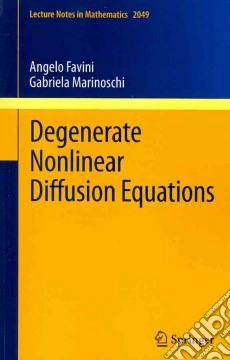ricerca
avanzata

# Degenerate Nonlinear Diffusion Equations

## Un libro in lingua di Angelo FaviniGabriela Marinoschi edito da Springer Verlag, 2012

• € 48,40
• Il prezzo è variabile in funzione del cambio della valuta d’origineThe aim of these notes is to include in a uniform presentation style several topics related to the theory of degenerate nonlinear diffusion equations, treated in the mathematical framework of evolution equations with multivalued m-accretive operators in Hilbert spaces. The problems concern nonlinear parabolic equations involving two cases of degeneracy. More precisely, one case is due to the vanishing of the time derivative coefficient and the other is provided by the vanishing of the diffusion coefficient on subsets of positive measure of the domain.From the mathematical point of view the results presented in these notes can be considered as general results in the theory of degenerate nonlinear diffusion equations. However, this work does not seek to present an exhaustive study of degenerate diffusion equations, but rather to emphasize some rigorous and efficient techniques for approaching various problems involving degenerate nonlinear diffusion equations, such as well-posedness, periodic solutions, asymptotic behaviour, discretization schemes, coefficient identification, and to introduce relevant solving methods for each of them.